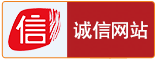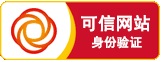2020-06-16 12:28

1、长方形的周长=(长+宽)×2 C=(a+b)×2

2、正方形的周长=边长×4 C=4a

3、长方形的面积=长×宽S=ab

4、正方形的面积=边长×边长S=a.a=a

5、三角形的面积=底×高÷2 S=ah÷2

6、平行四边形的面积=底×高S=ah

7、梯形的面积=(上底+下底)×高÷2 S=(a+b)h÷2

8、直径=半径×2 d=2r半径=直径÷2 r=d÷2

9、圆的周长=圆周率×直径=圆周率×半径×2 c=πd=2πr

10、圆的面积=圆周率×半径×半径

11、三角形的面积=底×高÷2。公式S=a×h÷2

12、正方形的面积=边长×边长公式S=a×a

13、长方形的面积=长×宽公式S=a×b

14、平行四边形的面积=底×高公式S=a×h

15、梯形的面积=(上底+下底)×高÷2公式S=(a+b)h÷2

16、内角和：三角形的内角和=180度。

17、长方体的体积=长×宽×高公式：V=abh

18、长方体(或正方体)的体积=底面积×高公式：V=abh

19、正方体的体积=棱长×棱长×棱长公式：V=aaa

20、圆的周长=直径×π公式：L=πd=2πr

21、圆的面积=半径×半径×π公式：S=πr2

22、圆柱的表(侧)面积：圆柱的表(侧)面积等于底面的周长乘高。公式：S=ch=πdh=2πrh

23、圆柱的表面积：圆柱的表面积等于底面的周长乘高再加上两头的圆的面积。公式：S=ch+2s=ch+2πr2

24、圆柱的体积：圆柱的体积等于底面积乘高。公式：V=Sh

25、圆锥的体积=1/3底面×积高。公式：V=1/3Sh

26、分数的加、减法则：同分母的分数相加减，只把分子相加减，分母不变。异分母的分数相加减，先通分，然后再加减。

分数的乘法则：用分子的积做分子，用分母的积做分母。

分数的除法则：除以一个数等于乘以这个数的倒数。